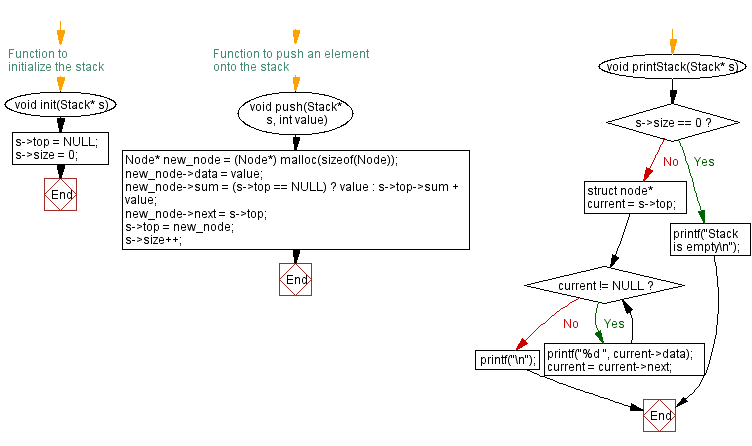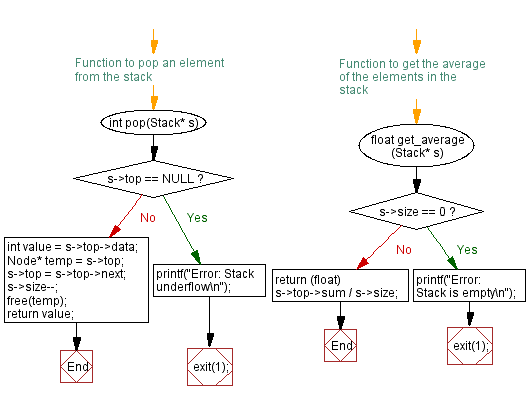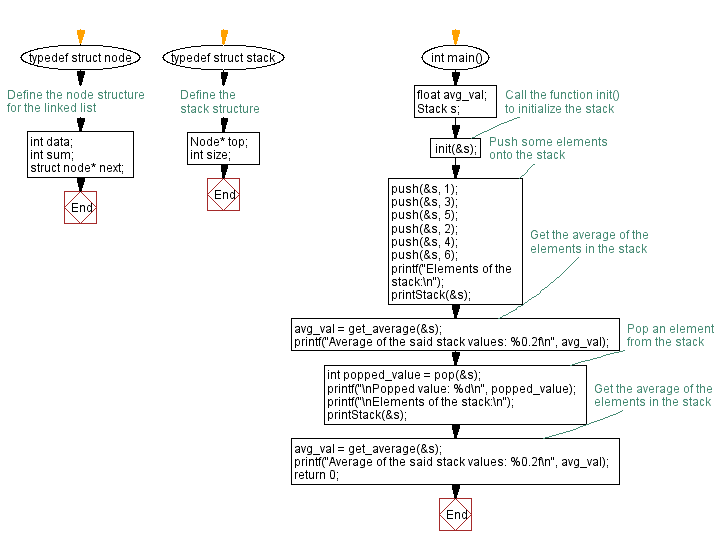﻿ C - Average value of the stack elements

# C Exercises: Average value of the stack elements

## C Stack: Exercise-14 with Solution

Write a C program to calculate the average value of the stack elements.

Sample Solution:

C Code:

``````#include <stdio.h>
#include <stdlib.h>

// Define the node structure for the linked list
typedef struct node {
int data;
int sum;
struct node* next;
} Node;

// Define the stack structure
typedef struct stack {
Node* top;
int size;
} Stack;

// Function to initialize the stack
void init(Stack* s) {
s->top = NULL;
s->size = 0;
}

// Function to push an element onto the stack
void push(Stack* s, int value) {
Node* new_node = (Node*) malloc(sizeof(Node));
new_node->data = value;
new_node->sum = (s->top == NULL) ? value : s->top->sum + value;
new_node->next = s->top;
s->top = new_node;
s->size++;
}

// Function to pop an element from the stack
int pop(Stack* s) {
if (s->top == NULL) {
printf("Error: Stack underflow\n");
exit(1);
}
int value = s->top->data;
Node* temp = s->top;
s->top = s->top->next;
s->size--;
free(temp);
return value;
}

// Function to get the average of the elements in the stack
float get_average(Stack* s) {
if (s->size == 0) {
printf("Error: Stack is empty\n");
exit(1);
}
return (float) s->top->sum / s->size;
}

void printStack(Stack* s) {
if (s->size == 0) {
printf("Stack is empty\n");
return;
}

struct node* current = s->top;
while (current != NULL) {
printf("%d ", current->data);
current = current->next;
}
printf("\n");
}

int main() {
float avg_val;
Stack s;
// Call the function init() to initialize the stack
init(&s);

// Push some elements onto the stack
push(&s, 1);
push(&s, 3);
push(&s, 5);
push(&s, 2);
push(&s, 4);
push(&s, 6);
printf("Elements of the stack:\n");
printStack(&s);
// Get the average of the elements in the stack
avg_val = get_average(&s);
printf("Average of the said stack values: %0.2f\n", avg_val);
// Pop an element from the stack
int popped_value = pop(&s);
printf("\nPopped value: %d\n", popped_value);
printf("\nElements of the stack:\n");
printStack(&s);
// Get the average of the elements in the stack
avg_val = get_average(&s);
printf("Average of the said stack values: %0.2f\n", avg_val);
return 0;
}
```
```

Sample Output:

```Elements of the stack:
6 4 2 5 3 1
Average of the said stack values: 3.50

Popped value: 6

Elements of the stack:
4 2 5 3 1
Average of the said stack values: 3.00
```

Flowchart:C Programming Code Editor:

Have another way to solve this solution? Contribute your code (and comments) through Disqus.

What is the difficulty level of this exercise?

Test your Programming skills with w3resource's quiz.

﻿

## C Programming: Tips of the Day

C Programming - How do you pass a function as a parameter in C?

Declaration

A prototype for a function which takes a function parameter looks like the following:

```void func ( void (*f)(int) );
```

This states that the parameter f will be a pointer to a function which has a void return type and which takes a single int parameter. The following function (print) is an example of a function which could be passed to func as a parameter because it is the proper type:

```void print ( int x ) {
printf("%d\n", x);
}
```

Function Call

When calling a function with a function parameter, the value passed must be a pointer to a function. Use the function's name (without parentheses) for this:

```func(print);
```

would call func, passing the print function to it.

Function Body

As with any parameter, func can now use the parameter's name in the function body to access the value of the parameter. Let's say that func will apply the function it is passed to the numbers 0-4. Consider, first, what the loop would look like to call print directly:

```for ( int ctr = 0 ; ctr < 5 ; ctr++ ) {
print(ctr);
}
```

Since func's parameter declaration says that f is the name for a pointer to the desired function, we recall first that if f is a pointer then *f is the thing that f points to (i.e. the function print in this case). As a result, just replace every occurrence of print in the loop above with *f:

```void func ( void (*f)(int) ) {
for ( int ctr = 0 ; ctr < 5 ; ctr++ ) {
(*f)(ctr);
}
}
```

Ref : https://bit.ly/3skw9Um